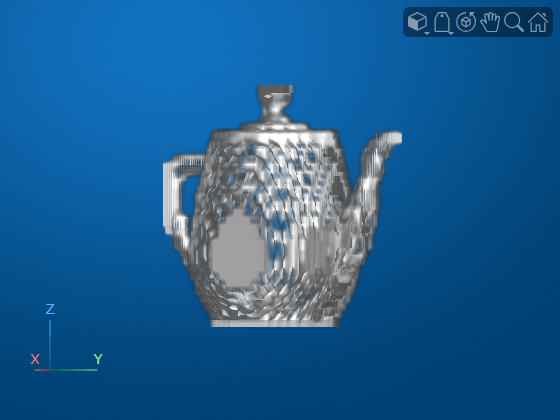# pcbin

Spatially bin point cloud points

## Syntax

``bins = pcbin(ptCloud,numBins)``
``bins = pcbin(ptCloud,numBins,spatialLimits)``
``[bins,binLocations] = pcbin(___)``
``[___] = pcbin(___,'BinOutput',true)``

## Description

example

````bins = pcbin(ptCloud,numBins)` spatially grids points in the point cloud, `ptCloud`. The output `bins` stores spatial bin locations, which are the indices for the points. The function finds the bin locations by sampling the point cloud in each dimension by the number of bins specified by `numBins`.```
````bins = pcbin(ptCloud,numBins,spatialLimits)` specifies the spatial limits to use when the function places points into bins.```
````[bins,binLocations] = pcbin(___)` also returns `binLocations`, the spatial bounds of each bin in `bins`. `binLocations` is the same size as `bins`.```
````[___] = pcbin(___,'BinOutput',true)` also specifies the format of the `bins` and `binLocations` output.When you set '`BinOutput`' to `true`, `bins` and `binLocations` are returned as cell arrays of size [numBinsX,numBinsY,numBinsZ].When you set '`BinOutput`' to `false` and `ptCloud` are unorganized point clouds, `bins` is returned as an M-by-1 numeric vector. `binLocations` is returned as an M-by-6 matrix.When you set '`BinOutput`' to `false` and `ptCloud` are organized point clouds, `bins` is returned an M-by-N matrix. `binLocations` is returned as an M-by-N-by-6 matrix.```

## Examples

collapse all

Read point cloud data into the workspace.

`ptCloud = pcread('teapot.ply');`

Spatially bin the point cloud into a 32-by-32-by-32 grid.

`indices = pcbin(ptCloud,[32 32 32]);`

Build an occupany grid.

`occupancyGrid = cellfun(@(c) ~isempty(c), indices);`

Display the occupany grid.

```ViewPnl = uipanel(figure); volshow(occupancyGrid,'Parent',ViewPnl);```Read point cloud data into the workspace.

`ptCloud = pcread('teapot.ply');`

Spatially bin the point cloud into a 32-by-32-by-1 grid.

`indices = pcbin(ptCloud,[32 32 1]);`

Build a density grid.

`densityGrid = cellfun(@(c) ~isempty(c),indices);`

Display the density grid.

```figure; imagesc(densityGrid);```## Input Arguments

collapse all

Point cloud, specified as a `pointCloud` object.

Number of bins, specified as a three-element vector of the form [numBinsX,numBinsY, numBinsZ]. The vector elements indicate the number of bins to use in each dimension, respectively.

Spatial limits, specified as a 3-by-2 numeric matrix of the form [Xmin, Xmax; Ymin, Ymax; Zmin, Zmax]. The spatial limits define the bounds used when the function bins points. Points that are outside of the specified limits are not included in the output.

## Output Arguments

collapse all

Spatial bin locations, returned as a cell array of the same size as input `numBins`, an M-by-1 numeric vector, or an M-by-N matrix. The function returns a cell array when you do not use the '`BinOutput`' argument. Each cell in the array contains indices to locations of points in the input point cloud that define the bins. Each bin includes the left bin edge, except for the last bin which includes both bin edges.

The table describes the size of `bins` based how you specify the '`BinOutput`' argument, and whether `ptCloud` is unorganized or organized.

'`BinOutput`'`bins`
`true`cell arrays of size [numBinsX,numBinsY,numBinsZ]
`false`

Unorganized point clouds

M-by-1 numeric vector

, where M represents the number of points.

Organized point clouds

M-by-N matrix, where M and N represents the total number of points set up as an organized point cloud.

The function processes invalid points differently, depending on how you specify the '`BinOutput`' argument. Invalid points are points with coordinates that contain `Inf` or `NaN` . When you set '`BinOutput`' to `true`, the function ignores invalid points and does not include them in the output. When you set '`BinOutput`' to `false`, the function returns `NaN` values for invalid points.

Bin spatial bounds, returned as a cell array the same size as the output `bins`, cell arrays of size [numBinsX,numBinsY,numBinsZ], an M-by-6 matrix, or an M-by-N-by-6 matrix . The function returns a cell array when you do not use the '`BinOutput`' argument. Each cell in the array contains the spatial bounds of the bins in `bins`. The bounds for a bin are of the form [Xmin, Xmax; Ymin, Ymax; Zmin, Zmax].

The table describes the size of `binLocations` based how you specify the '`BinOutput`' argument, and whether `ptCloud` is unorganized or organized.

'`BinOutput`'`binLocations`
`true`cell arrays of size [numBinsX,numBinsY,numBinsZ]
`false`

Unorganized point clouds:

M-by-6 matrix, where M represents the number of points located in the bin specified by the [Xmin, Xmax; Ymin, Ymax; Zmin, Zmax] spatial bound.

Organized point clouds:

M-by-N-by-6 matrix, where M and N represents the number of points in an organized point cloud, with each point located in the bin specified by the [Xmin, Xmax; Ymin, Ymax; Zmin, Zmax] spatial bound.

## Version History

Introduced in R2020a